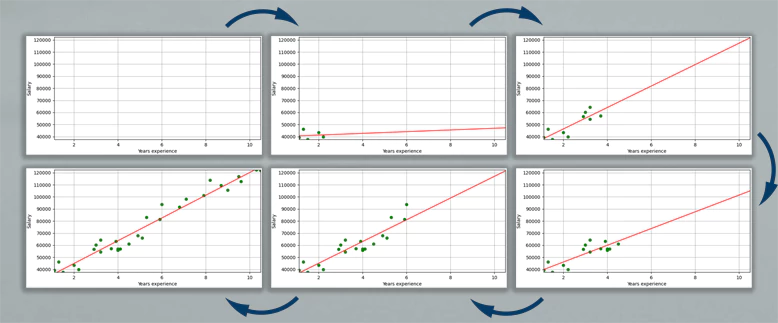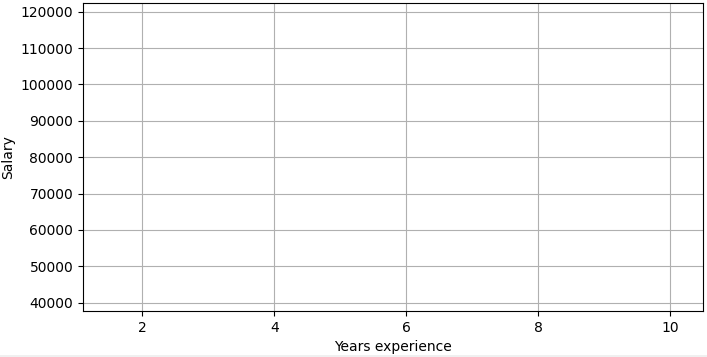# Animate Line Fitting of Simple Linear Regression using Matplotlib

Simple linear regression is a statistical method that is used to analyze the relationship between two continuous variables. During a line fitting process using linear regression, it can be useful to see how the line fits the data when more data are added. This tutorial provides code example how to create line fitting animation of simple linear regression.

## Prepare environment

• Install the following packages using `pip`:
``pip install scikit-learn``
``pip install matplotlib``
``pip install pandas``
• Download salary dataset from Kaggle and name it `salary.csv`. The dataset contains salary based on the years of experience.

## Code

The following steps are performed in the code:

• Using the `Pandas` library, we read a CSV file and load data into a `DataFrame`.
• We select two columns from `DataFrame` and assign values to variables `x` and `y` respectively, which will be used for the training process.
• We set the x-axis and y-axis view limits, labels and enable the grid lines.
• We initialize the `LinearRegression` model provided by the `scikit-learn` library.
• The `Matplotlib` library provides the `FuncAnimation` function, which repeatedly calls the `animate` function. The delay between frames is configured with `interval` parameter. In our case, 200 milliseconds.
• On each call of `animate` function, more data is added to the training set.
• We fit a linear regression model to training data and make predictions.
• We draw a scatter and line plots to visualize training set and predicted values.
``````import matplotlib.pyplot as plt
import numpy as np
import pandas as pd
from matplotlib.animation import FuncAnimation
from sklearn.linear_model import LinearRegression

x = df['YearsExperience'].to_numpy()
y = df['Salary'].to_numpy()

x_train = []
y_train = []
x_test = np.arange(x.min(), x.max() + 1).reshape(-1, 1)

fig, ax = plt.subplots(figsize=(8, 4))
ax.set_xlim(x.min(), x.max())
ax.set_ylim(y.min(), y.max())
ax.set_xlabel('Years experience')
ax.set_ylabel('Salary')
ax.grid()

scatter, = ax.plot([], [], 'go')
line, = ax.plot([], [], 'r')

lr = LinearRegression()

def animate(n):
x_train.append([x[n]])
y_train.append([y[n]])

lr.fit(x_train, y_train)
y_test = lr.predict(x_test)

scatter.set_data(x_train, y_train)
line.set_data(x_test, y_test)

anim = FuncAnimation(fig, animate, frames=x.size, interval=200, repeat=False)
plt.show()``````

## Result